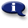# .

## Syllabus Information

Summer 2021
Dec 04,2021Use this page to maintain syllabus information, learning objectives, required materials, and technical requirements for the course.

Syllabus Information
MTH 020 - Math Renewal
Associated Term: Summer 2021
Learning Objectives: Upon successful completion of this course, the student should be able to: 1. Apply reasoning and problem solving skills to basic mathematics problems. 2. Use estimation in basic math problems. 3. Check the reasonableness of answers. 4. Perform fraction computations and applications with accuracy. 5. Perform decimal computations and applications with accuracy. 4. Write and simplify ratios and rates. 6. Recognize when and where ratios or proportions apply. 7. Set up and solve proportions. 8. Convert between fractions, decimals, and percents. 9. Solve the three basic types of percent problems. 10. Set up and solve percent application problems. 11. Solve applications using area and perimeter of simple geometric shapes. 12. Solve basic equations of the type a + x = b and ax = b, involving whole numbers, fractions and decimals. 13. Use a scientific calculator to explore and solve basic math problems. 14. Apply study skills for learning mathematics and for coping with math anxiety. 15. Use the symbols and vocabulary of basic mathematics correctly. 16. Use American and metric measurement.
Required Materials:
Technical Requirements: